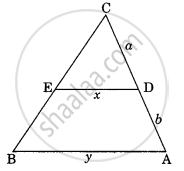# In the given figure DE || AC which of the following is true? - Mathematics

MCQ

In the given figure DE || AC which of the following is true?#### Options

• x = ("a + b")/"ay"

• y = "ax"/("a + b")

• x = "ay"/("a + b")

• x/y = "a"/"b"

#### Solution

x = "ay"/("a + b")

Concept: Areas of Similar Triangles
Is there an error in this question or solution?